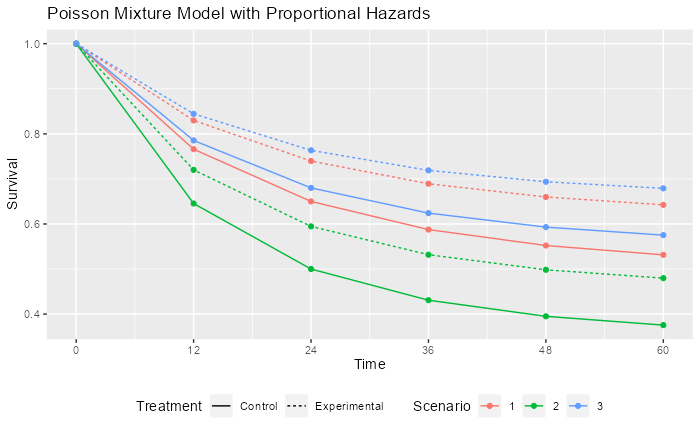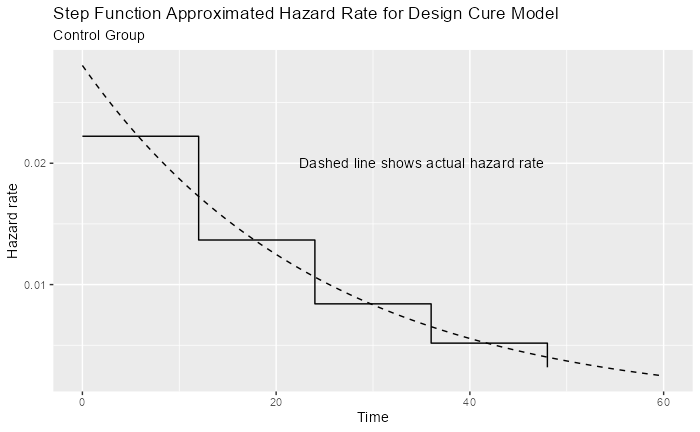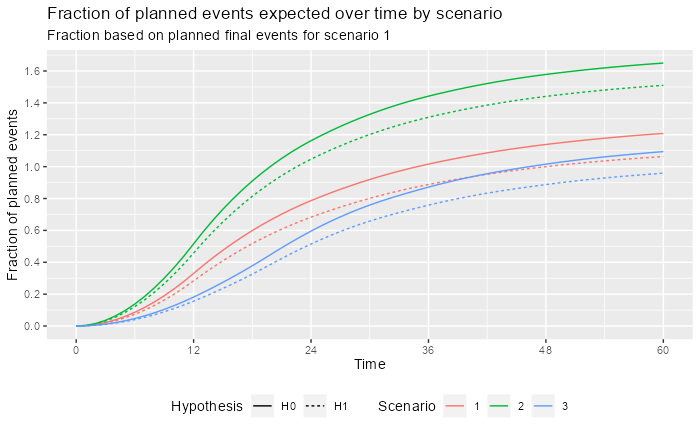# Introduction

We present a study design for a time-to-event outcome based on a cure model (Rodrigues et al. (2009)). In this case, it is assumed that tail behavior of the survival is of substantial interest and there is no desire to stop and do a final analysis before substantial follow-up through 4 years has been allowed to accrue. It is assumed further if substantial events have not accrued in this time period, then some sacrifice in power would not be unreasonable. Due to this as well as substantial variability in event accrual caused by feasible differences in event rates, we use a calendar-based design, including calendar-based spending (Lan and DeMets (1989)).

We discuss some of the potential advantages and disadvantages of the cure model and calendar-based design cases where hazard rates for events decrease substantially over time and the true underlying distributions may meaningfully deviate from what is anticipated at the time of design.

# The Poisson mixture model

The Poisson mixture model is a cure model that can be useful when the failure rate in a population is expected to decline substantially over time based on historical data. It also has the property that if control group time-to-event follows a Poisson mixture distribution, then a proportional hazards assumption for treatment effect will yield another Poisson mixture distribution for the experimental group. The model is flexible and easy to use in that the control distribution is specified with two parameters in a transparent fashion: the cure rate and one other survival rate at an arbitrarily specified time point.

## Scenario assumptions

We consider three scenarios to demonstrate how spending can impact potential for trial success and fully understanding treatment group differences. The following can be adjusted by the reader and the vignette re-run.

# Control group assumptions for three Poisson mixture cure models
cure_rate <- c(.5, .35, .55)
# Second time point for respective models
t1 <- c(24, 24, 24)
# Survival rate at 2nd time point for respective models
s1 <- c(.65, .5, .68)
time_unit <- "month"
# Hazard ratio for experimental versus control for respective models
hr <- c(.7, .75, .7)
# Total study duration
study_duration <- c(48, 48, 56)
# Number of bins for piecewise approximation
bins <- 5

We will assume a constant enrollment rate for the duration of enrollment, allowing different assumed enrollment durations by scenario. The following code can be easily changed to study alternate scenarios.

# This code should be updated by user for their scenario
# Enrollment duration by scenario
enroll_duration <- c(12, 12, 20)
# Dropout rate (exponential failure rate per time unit) by scenario
dropout_rate <- c(.002, .001, .001)

## The Poisson Mixture Model

The Poisson mixture model (Rodrigues et al. (2009)) assumes a cure rate $$p$$ to represent the patients who benefit long-term. The survival function as a function of time $$t$$ for a control group ($$c$$) is:

$S_c(t)=\exp(-\theta(1-\exp(-\lambda t))),$ where $$\theta = -\log(p)$$, $$\lambda> 0$$ is a constant hazard rate and $$t\ge 0$$. The component $$\exp(-\lambda t)$$ is an exponential survival distribution; while it could be replaced with an arbitrary survival distribution on $$t>0$$ for the mixture model, the exponential model is simple, adequately flexible and easy to explain. This two-parameter model can be specified by the cure rate and the assumed survival rate $$S_c(t_1)$$ at some time $$0 <t_1<\infty.$$ For this study, the control group cure rate is assumed to be 0.5, 0.35, 0.55 and the survival at month is assumed to be 0.65, 0.5, 0.68. We can solve for $$\theta$$ and $$\lambda$$ as follows:

$S_c(\infty) = e^\theta \Rightarrow \theta = -\log(S_c(\infty))$ and with a little algebra, we can solve for $$\lambda$$: $S_c(t_1)= \exp(-\theta(1-\exp(-\lambda t_1))) \Rightarrow \lambda = -\log(1 + \log(S_c(t_1)) / \theta) / t_1$

## Supporting functions

We create the following functions to support examples below.

• pPM() computes a Poisson mixture survival function
• hPM() computes Poisson mixture hazard rates

Most readers should skip reviewing this code.

# Poisson mixture survival
pPM <- function(x = 0:20, cure_rate = .5, t1 = 10, s1 = .6) {
theta <- -log(cure_rate)
lambda <- -log(1 + log(s1) / theta) / t1
return(exp(-theta * (1 - exp(-lambda * x))))
}
# Poisson mixture hazard rate
hPM <- function(x = 0:20, cure_rate = .5, t1 = 10, s1 = .6) {
theta <- -log(cure_rate)
lambda <- -log(1 + log(s1) / theta) / t1
return(theta * lambda * exp(-lambda * x))
}

## Examples

We note that under a proportional hazards assumption with hazard ratio $$\gamma > 0$$ the survival funtion for the experimental group (e) is:

$S_e(t)=\exp(-\theta\gamma(1-\exp(-\lambda t))).$ As noted before, $$(1 - \exp(-\lambda t)$$ can be replaced with the cumulative distribution for any positive random variable. For any setting chosen, it is ideal to be able to cite published literature and other rationale for study assumptions.

The points in the following graph indicate where underlying cumulative hazard matches the piecewise exponential of the specified cure rate models by scenario. The piecewise failure model is used to derive the sample size and targeted events over time in the trial.We also evaluate the failure rate over time, which is higher in scenario 2. This is later used in the design derivation. Note that the piecewise intervals used to approximate changing hazard rates can be made arbitrarily small to get more precise approximations of the above. However, given the uncertainty of the underlying assumptions, it is not clear that this provides any advantage.## Event Accumulation

Based on the above model, we predict how events will accumulate for the control group, experimental group under the alternate hypothesis and overall based on either the null hypothesis of no failure rate difference or the alternate hypothesis where events accrue more slowly in the experimental group. We do this by scenario. We use as a denominator the final planned events under the alternate hypothesis for scenario 1.

Now we compare event accrual under the null and alternate hypothesis for each scenario, with 100% representing the targeted final events under scenario 1. The user should not have to update the code here. For the 3 scenarios studied, event accrual is quite different, creating difference spending issues.# Study design

## Design assumptions

We choose calendar-based timing for analyses as well as for spending. This is not done automatically by the gsSurv() function. There are two steps in particular that we walk through here:

• How to get information fraction levels that correspond to targeted calendar analysis times to plug in for the planned design.
• Replacing information fraction levels with calendar fraction levels for $$\alpha$$- and $$\beta$$-spending.

We begin by specifying calendar times of analysis and find corresponding fractions of final planned events and calendar time under design assumptions.

h1s1_EF <- event_accrual %>%
filter(Scenario == 1 & Hypothesis == "H1" & Time %in% c(12, 24, 36)) %>%
select(Time, EF)
h1s1_EF
#> # A tibble: 3 × 2
#>    Time    EF
#>   <dbl> <dbl>
#> 1    12 0.284
#> 2    24 0.683
#> 3    36 0.888
# Interim analysis timing (information fraction)
timing <- h1s1_EF$EF timing_calendar <- h1s1_EF$Time / study_duration

Now we move on to other design assumptions.

# Get hazard rate info for Scenario 1 control group
control <- hazard %>% filter(Scenario == 1, Treatment == "Control")
# Failure rates
lambdaC <- control$hazard_rate # Interval durations S <- (control$Time - control\$time_lagged)[1:(bins - 1)]
# 1-sided Type I error
alpha <- 0.025
# Type II error (1 - power)
beta <- .1
# Test type 6: asymmetric 2-sided design, non-binding futility bound
test.type <- 6
# 1-sided Type I error used for safety (for asymmetric 2-sided design)
astar <- .2
# Spending functions (sfu, sfl) and parameters (sfupar, sflpar)
sfu <- sfHSD # O'Brien-Fleming approximation by Lan and DeMets
sfupar <- -4 # Not needed for sfLDPocock
sfl <- sfLDPocock # Near-equal Z-values for each analysis
sflpar <- NULL # Not needed for Pocock spending
# Dropout rate (exponential parameter per unit of time)
dropout_rate <- 0.002
# Experimental / control randomization ratio
ratio <- 1

## Study Design and Event Accumulation

We now assume a trial is enrolled with a constant enrollment rate over 12, 12, 20 months trial duration of 48, 48, 56. As noted above, the event accumulation pattern is highly sensitive to the assumptions of the design. Deviations from plan in accrual, the hazard ratio overall or over time as well as relatively minor deviations from the cure model assumption could substantially change the calendar time of event-based analysis timing. Thus, calendar-based timing and spending (Lan and DeMets (1989)) may have some appeal to make the timing of analyses more predictable. The main risk to this would likely be under-accumulation of the final targeted events for the trial. The targeted 4-year window may be considered clinically important as well as an important limitation for trial duration. Using the above predicted information fractions at 6, 12, 24, 36, 48, and 60 months to plan a calendar-based design. We use the arguments usTime and lsTime to change to calendar-based spending for the upper and lower bounds, respectively. However, the pattern of slowing event accumulation over time after year 1 seems reasonably likely to persist. This means that calendar-based spending is likely to give more conservative bounds since the calendar fractions are lower than the information fractions in the text overlay of the plot after the first interim: 10%, 20%, 40%, 60%, 80% and 100%, respectively.

We now use the information fractions from the text overlay to set up a calendar-based design.

design_calendar <-
gsSurv(
k = length(timing) + 1,
alpha = alpha,
beta = beta,
astar = astar,
test.type = test.type,
timing = timing, # Planned event fractions here
hr = hr,
R = enroll_duration,
gamma = 1,
T = study_duration,
minfup = study_duration - enroll_duration,
ratio = ratio,
sfu = sfu,
sfupar = sfupar,
usTime = timing_calendar, # Use calendar-based spending
sfl = sfl,
sflpar = sflpar,
lambdaC = lambdaC,
lsTime = timing_calendar, # Use calendar-based spending
S = S
)
design_calendar %>%
gsBoundSummary(exclude = c("B-value", "CP", "CP H1", "PP")) %>%
gt() %>%
title = "Calendar-Based Design",
subtitle = "Calendar Spending"
)
Analysis Value Efficacy Futility Calendar-Based Design Calendar Spending IA 1: 28% Z 3.1554 -1.4649 N: 884 p (1-sided) 0.0008 0.9285 Events: 95 ~HR at bound 0.5228 1.3513 Month: 12 Spending 0.0008 0.0715 P(Cross) if HR=1 0.0008 0.0715 P(Cross) if HR=0.7 0.0773 0.0007 IA 2: 68% Z 2.8268 -1.3883 N: 884 p (1-sided) 0.0024 0.9175 Events: 229 ~HR at bound 0.6877 1.2019 Month: 24 Spending 0.0022 0.0525 P(Cross) if HR=1 0.0030 0.1240 P(Cross) if HR=0.7 0.4516 0.0007 IA 3: 89% Z 2.6510 -1.3943 N: 884 p (1-sided) 0.0040 0.9184 Events: 297 ~HR at bound 0.7350 1.1758 Month: 36 Spending 0.0027 0.0248 P(Cross) if HR=1 0.0056 0.1488 P(Cross) if HR=0.7 0.6761 0.0007 Final Z 1.9749 -1.0483 N: 884 p (1-sided) 0.0241 0.8527 Events: 334 ~HR at bound 0.8056 1.1216 Month: 48 Spending 0.0194 0.0512 P(Cross) if HR=1 0.0250 0.2000 P(Cross) if HR=0.7 0.9000 0.0007

# Considerations

There are a few things to note for the above design:

• The futility bounds are advisory only. In particular, the late futility bounds may be ignored since the follow-up for the full time period may merit continuing the trial.
• Substantial deviations in event accumulation would not change timing of analyses from their calendar times. This should be considered for acceptability at the time of design.
• The first efficacy bound is so extreme that it essentially makes the first analysis futility only. This is likely reasonable based on the minimal follow-up at that time.
• The Z-values and hazard ratios required for a positive efficacy finding are not terribly extreme starting from the two-year follow-up analysis. This is also probably reasonable as long as the hazard ratio differences at the bounds are clinically meaningful. A more extreme finding at year 1 may likely be required for a positive finding.
• The trial may be continued after crossing an efficacy bound for further follow-up as it is unlikely that control patients doing well would cross over to experimental therapy in absence of adverse clinical outcomes. Inference at subsequent analyses using repeated p-values (Jennison and Turnbull (2000)) or sequential p-values (Liu and Anderson (2008)) are well-specified and interpretable as adjusted p-values.